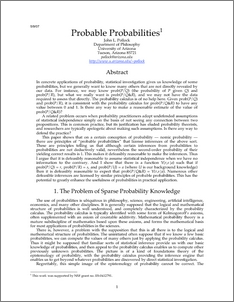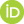# Probable Probabilities

Pollock, John (2007) Probable Probabilities. [Preprint]Preview
PDF
Probable_Probabilities.pdf

## Abstract

In concrete applications of probability, statistical investigation gives us knowledge of some probabilities, but we generally want to know many others that are not directly revealed by our data. For instance, we may know prob(P/Q) (the probability of P given Q) and prob(P/R), but what we really want is prob(P/Q&R), and we may not have the data required to assess that directly. The probability calculus is of no help here. Given prob(P/Q) and prob(P/R), it is consistent with the probability calculus for prob(P/Q&R) to have any value between 0 and 1. Is there any way to make a reasonable estimate of the value of prob(P/Q&R)? A related problem occurs when probability practitioners adopt undefended assumptions of statistical independence simply on the basis of not seeing any connection between two propositions. This is common practice, but its justification has eluded probability theorists, and researchers are typically apologetic about making such assumptions. Is there any way to defend the practice? This paper shows that on a certain conception of probability — nomic probability — there are principles of “probable probabilities” that license inferences of the above sort. These are principles telling us that although certain inferences from probabilities to probabilities are not deductively valid, nevertheless the second-order probability of their yielding correct results is 1. This makes it defeasibly reasonable to make the inferences. Thus I argue that it is defeasibly reasonable to assume statistical independence when we have no information to the contrary. And I show that there is a function Y(r,s:a) such that if prob(P/Q) = r, prob(P/R) = s, and prob(P/U) = a (where U is our background knowledge) then it is defeasibly reasonable to expect that prob(P/Q&R) = Y(r,s:a). Numerous other defeasible inferences are licensed by similar principles of probable probabilities. This has the potential to greatly enhance the usefulness of probabilities in practical application.

 Export/Citation: EndNote | BibTeX | Dublin Core | ASCII/Text Citation (Chicago) | HTML Citation | OpenURL
 Social Networking:

Item Type: Preprint
Creators:
CreatorsEmailORCIDPollock, John
Keywords: objective probability, nomic probability, direct inference, statistical syllogism, defeasible reasoning
Subjects: Specific Sciences > Probability/Statistics
General Issues > Decision Theory
Depositing User: John L. Pollock
Date Deposited: 19 Jun 2007
Item ID: 3340
Subjects: Specific Sciences > Probability/Statistics
General Issues > Decision Theory
Date: May 2007
URI: http://philsci-archive.pitt.edu/id/eprint/3340

### Monthly Views for the past 3 yearsView Item